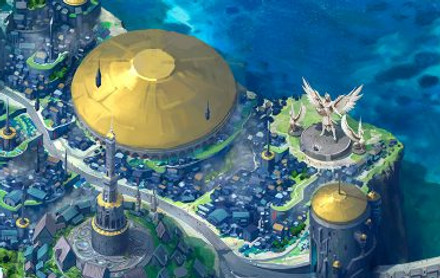top of page

### The quests are listed in order of the story. They are designed for standard mastery so each quest covers different topics under each standard. To follow the story, the quests must be completed in order.4.N.1 Solve real-world and mathematical problems using multiplication and division.

4.N.2 Represent and compare fractions and decimals in real-world and mathematical situations; use place value to understand how decimals represent quantities.

4.N.3 Determine the value of coins in order to solve monetary transactions.

4.A.1 Use multiple representations of patterns to solve real-world and mathematical problems.

4.A.2 Use multiplication and division with unknowns to create number sentences representing a given problem situation

4.D.1 Collect, organize, and analyze data.

4.GM.1 Name, describe, classify and construct polygons, and three dimensional figures.

4.GM.2 Understand angle, length, and area as measurable attributes of real world and mathematical objects. Use various tools to measure angles, length, area, and volume.

4.GM.3 Determine elapsed time and convert between units of time.

bottom of page